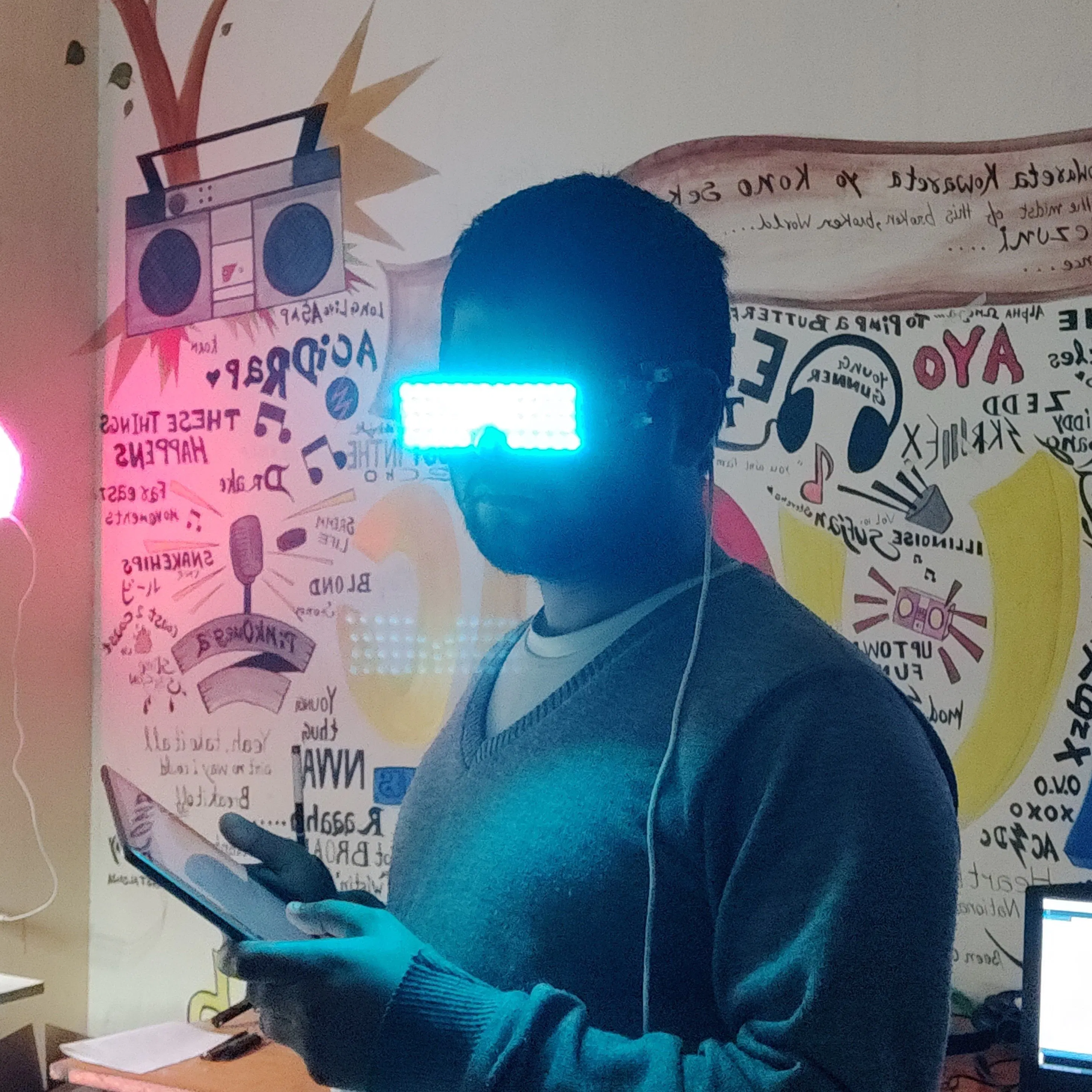# Minimal Arduino Uno

ATmega328P standalone/minimal setup.

IntermediateFull instructions provided18 minutes2,959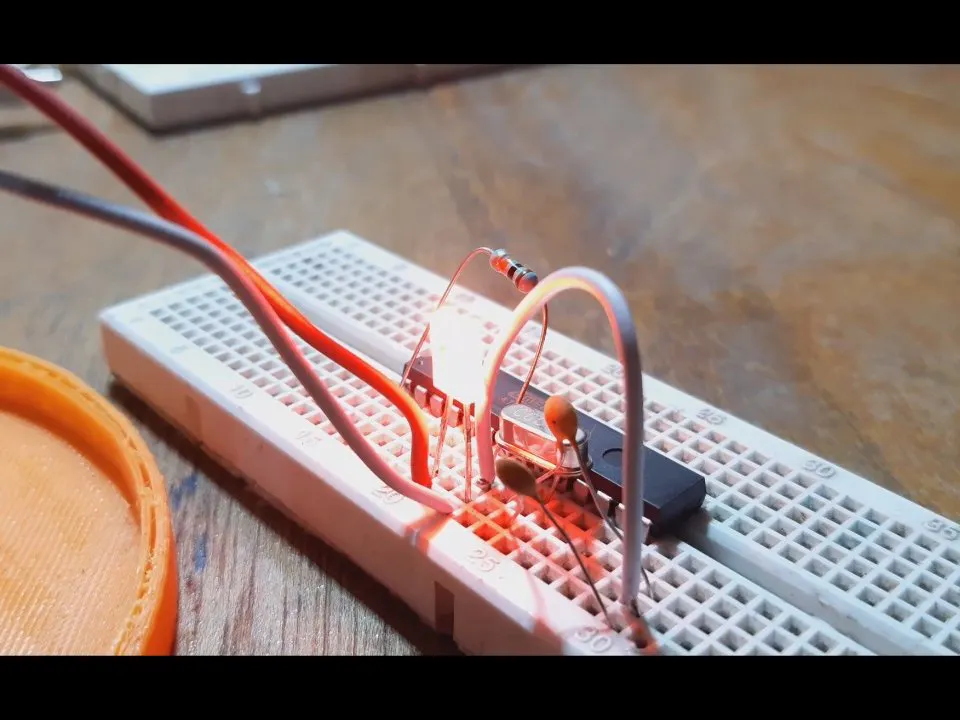## Things used in this project

### Hardware componentsArduino Nano R3
×1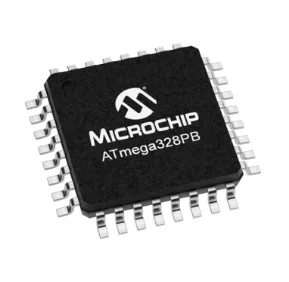Microchip Technology ATmega328PB microcontroller
×1
 16mhz crystal
×1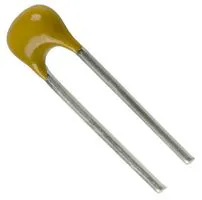Capacitor 22 pF
×1
 10uf capacitor
×1UTSOURCE Electronic Parts everything above can be found here for a low price
×1

### Software apps and online servicesArduino IDE

## Schematics

### scheme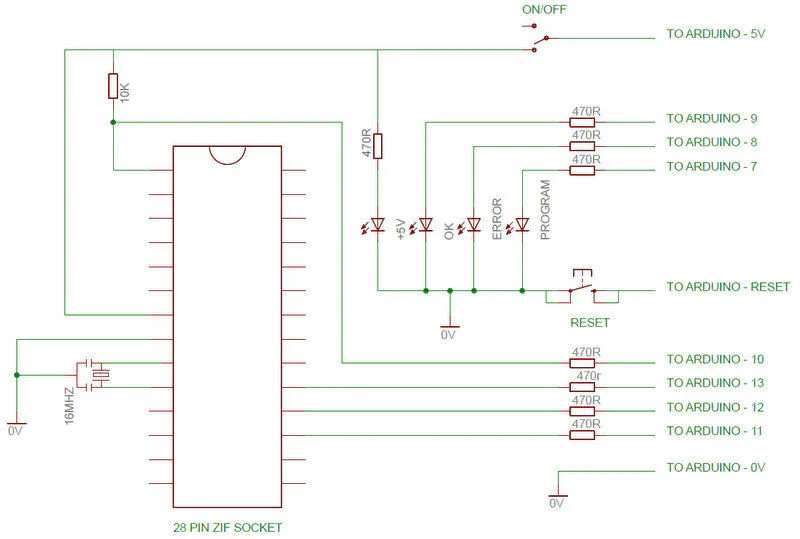## Code

### Arduino as ISP

C/C++
```// ArduinoISP
// Copyright (c) 2008-2011 Randall Bohn
// If you require a license, see
//
// This sketch turns the Arduino into a AVRISP using the following Arduino pins:
//
// Pin 10 is used to reset the target microcontroller.
//
// By default, the hardware SPI pins MISO, MOSI and SCK are used to communicate
// with the target. On all Arduinos, these pins can be found
//
//               MISO °. . 5V (!) Avoid this pin on Due, Zero...
//               SCK   . . MOSI
//                     . . GND
//
// On some Arduinos (Uno,...), pins MOSI, MISO and SCK are the same pins as
// digital pin 11, 12 and 13, respectively. That is why many tutorials instruct
// you to hook up the target to these pins. If you find this wiring more
// practical, have a define USE_OLD_STYLE_WIRING. This will work even when not
// using an Uno. (On an Uno this is not needed).
//
// Alternatively you can use any other digital pin by configuring
// software ('BitBanged') SPI and having appropriate defines for PIN_MOSI,
// PIN_MISO and PIN_SCK.
//
// IMPORTANT: When using an Arduino that is not 5V tolerant (Due, Zero, ...) as
// the programmer, make sure to not expose any of the programmer's pins to 5V.
// A simple way to accomplish this is to power the complete system (programmer
// and target) at 3V3.
//
// Put an LED (with resistor) on the following pins:
// 9: Heartbeat   - shows the programmer is running
// 8: Error       - Lights up if something goes wrong (use red if that makes sense)
// 7: Programming - In communication with the slave
//

#include "Arduino.h"
#undef SERIAL

#define PROG_FLICKER true

// Configure SPI clock (in Hz).
// E.g. for an ATtiny @ 128 kHz: the datasheet states that both the high and low
// SPI clock pulse must be > 2 CPU cycles, so take 3 cycles i.e. divide target
// f_cpu by 6:
//     #define SPI_CLOCK            (128000/6)
//
// A clock slow enough for an ATtiny85 @ 1 MHz, is a reasonable default:

#define SPI_CLOCK 		(1000000/6)

// Select hardware or software SPI, depending on SPI clock.
// Currently only for AVR, for other architectures (Due, Zero,...), hardware SPI
// is probably too fast anyway.

#if defined(ARDUINO_ARCH_AVR)

#if SPI_CLOCK > (F_CPU / 128)
#define USE_HARDWARE_SPI
#endif

#endif

// Configure which pins to use:

// The standard pin configuration.

#define RESET     10 // Use pin 10 to reset the target rather than SS
#define LED_HB    9
#define LED_ERR   8
#define LED_PMODE 7

// Uncomment following line to use the old Uno style wiring
// (using pin 11, 12 and 13 instead of the SPI header) on Leonardo, Due...

// #define USE_OLD_STYLE_WIRING

#ifdef USE_OLD_STYLE_WIRING

#define PIN_MOSI	11
#define PIN_MISO	12
#define PIN_SCK		13

#endif

// HOODLOADER2 means running sketches on the ATmega16U2 serial converter chips
// on Uno or Mega boards. We must use pins that are broken out:
#else

#define RESET     	4
#define LED_HB    	7
#define LED_ERR   	6
#define LED_PMODE 	5

#endif

// By default, use hardware SPI pins:
#ifndef PIN_MOSI
#define PIN_MOSI 	MOSI
#endif

#ifndef PIN_MISO
#define PIN_MISO 	MISO
#endif

#ifndef PIN_SCK
#define PIN_SCK 	SCK
#endif

// Force bitbanged SPI if not using the hardware SPI pins:
#if (PIN_MISO != MISO) ||  (PIN_MOSI != MOSI) || (PIN_SCK != SCK)
#undef USE_HARDWARE_SPI
#endif

// Configure the serial port to use.
//
// Prefer the USB virtual serial port (aka. native USB port), if the Arduino has one:
//   - it does not autoreset (except for the magic baud rate of 1200).
//   - it is more reliable because of USB handshaking.
//
// Leonardo and similar have an USB virtual serial port: 'Serial'.
// Due and Zero have an USB virtual serial port: 'SerialUSB'.
//
// On the Due and Zero, 'Serial' can be used too, provided you disable autoreset.
// To use 'Serial': #define SERIAL Serial

#ifdef SERIAL_PORT_USBVIRTUAL
#define SERIAL SERIAL_PORT_USBVIRTUAL
#else
#define SERIAL Serial
#endif

// Configure the baud rate:

#define BAUDRATE	19200
// #define BAUDRATE	115200
// #define BAUDRATE	1000000

#define HWVER 2
#define SWMAJ 1
#define SWMIN 18

// STK Definitions
#define STK_OK      0x10
#define STK_FAILED  0x11
#define STK_UNKNOWN 0x12
#define STK_INSYNC  0x14
#define STK_NOSYNC  0x15
#define CRC_EOP     0x20 //ok it is a space...

void pulse(int pin, int times);

#ifdef USE_HARDWARE_SPI
#include "SPI.h"
#else

#define SPI_MODE0 0x00

class SPISettings {
public:
// clock is in Hz
SPISettings(uint32_t clock, uint8_t bitOrder, uint8_t dataMode) : clock(clock) {
(void) bitOrder;
(void) dataMode;
};

private:
uint32_t clock;

friend class BitBangedSPI;
};

class BitBangedSPI {
public:
void begin() {
digitalWrite(PIN_SCK, LOW);
digitalWrite(PIN_MOSI, LOW);
pinMode(PIN_SCK, OUTPUT);
pinMode(PIN_MOSI, OUTPUT);
pinMode(PIN_MISO, INPUT);
}

void beginTransaction(SPISettings settings) {
pulseWidth = (500000 + settings.clock - 1) / settings.clock;
if (pulseWidth == 0)
pulseWidth = 1;
}

void end() {}

uint8_t transfer (uint8_t b) {
for (unsigned int i = 0; i < 8; ++i) {
digitalWrite(PIN_MOSI, (b & 0x80) ? HIGH : LOW);
digitalWrite(PIN_SCK, HIGH);
delayMicroseconds(pulseWidth);
b = (b << 1) | digitalRead(PIN_MISO);
digitalWrite(PIN_SCK, LOW); // slow pulse
delayMicroseconds(pulseWidth);
}
return b;
}

private:
unsigned long pulseWidth; // in microseconds
};

static BitBangedSPI SPI;

#endif

void setup() {
SERIAL.begin(BAUDRATE);

pinMode(LED_PMODE, OUTPUT);
pulse(LED_PMODE, 2);
pinMode(LED_ERR, OUTPUT);
pulse(LED_ERR, 2);
pinMode(LED_HB, OUTPUT);
pulse(LED_HB, 2);

}

int error = 0;
int pmode = 0;
unsigned int here;
uint8_t buff; // global block storage

typedef struct param {
uint8_t devicecode;
uint8_t revision;
uint8_t progtype;
uint8_t parmode;
uint8_t polling;
uint8_t selftimed;
uint8_t lockbytes;
uint8_t fusebytes;
uint8_t flashpoll;
uint16_t eeprompoll;
uint16_t pagesize;
uint16_t eepromsize;
uint32_t flashsize;
}
parameter;

parameter param;

// this provides a heartbeat on pin 9, so you can tell the software is running.
uint8_t hbval = 128;
int8_t hbdelta = 8;
void heartbeat() {
static unsigned long last_time = 0;
unsigned long now = millis();
if ((now - last_time) < 40)
return;
last_time = now;
if (hbval > 192) hbdelta = -hbdelta;
if (hbval < 32) hbdelta = -hbdelta;
hbval += hbdelta;
analogWrite(LED_HB, hbval);
}

static bool rst_active_high;

void reset_target(bool reset) {
digitalWrite(RESET, ((reset && rst_active_high) || (!reset && !rst_active_high)) ? HIGH : LOW);
}

void loop(void) {
// is pmode active?
if (pmode) {
digitalWrite(LED_PMODE, HIGH);
} else {
digitalWrite(LED_PMODE, LOW);
}
// is there an error?
if (error) {
digitalWrite(LED_ERR, HIGH);
} else {
digitalWrite(LED_ERR, LOW);
}

// light the heartbeat LED
heartbeat();
if (SERIAL.available()) {
avrisp();
}
}

uint8_t getch() {
while (!SERIAL.available());
}
void fill(int n) {
for (int x = 0; x < n; x++) {
buff[x] = getch();
}
}

#define PTIME 30
void pulse(int pin, int times) {
do {
digitalWrite(pin, HIGH);
delay(PTIME);
digitalWrite(pin, LOW);
delay(PTIME);
} while (times--);
}

void prog_lamp(int state) {
if (PROG_FLICKER) {
digitalWrite(LED_PMODE, state);
}
}

uint8_t spi_transaction(uint8_t a, uint8_t b, uint8_t c, uint8_t d) {
SPI.transfer(a);
SPI.transfer(b);
SPI.transfer(c);
return SPI.transfer(d);
}

if (CRC_EOP == getch()) {
SERIAL.print((char)STK_INSYNC);
SERIAL.print((char)STK_OK);
} else {
error++;
SERIAL.print((char)STK_NOSYNC);
}
}

if (CRC_EOP == getch()) {
SERIAL.print((char)STK_INSYNC);
SERIAL.print((char)b);
SERIAL.print((char)STK_OK);
} else {
error++;
SERIAL.print((char)STK_NOSYNC);
}
}

void get_version(uint8_t c) {
switch (c) {
case 0x80:
break;
case 0x81:
break;
case 0x82:
break;
case 0x93:
break;
default:
}
}

void set_parameters() {
// call this after reading parameter packet into buff[]
param.devicecode = buff;
param.revision   = buff;
param.progtype   = buff;
param.parmode    = buff;
param.polling    = buff;
param.selftimed  = buff;
param.lockbytes  = buff;
param.fusebytes  = buff;
param.flashpoll  = buff;
// ignore buff (= buff)
// following are 16 bits (big endian)
param.eeprompoll = beget16(&buff);
param.pagesize   = beget16(&buff);
param.eepromsize = beget16(&buff);

// 32 bits flashsize (big endian)
param.flashsize = buff * 0x01000000
+ buff * 0x00010000
+ buff * 0x00000100
+ buff;

// AVR devices have active low reset, AT89Sx are active high
rst_active_high = (param.devicecode >= 0xe0);
}

void start_pmode() {

// Reset target before driving PIN_SCK or PIN_MOSI

// SPI.begin() will configure SS as output, so SPI master mode is selected.
// We have defined RESET as pin 10, which for many Arduinos is not the SS pin.
// So we have to configure RESET as output here,
// (reset_target() first sets the correct level)
reset_target(true);
pinMode(RESET, OUTPUT);
SPI.begin();
SPI.beginTransaction(SPISettings(SPI_CLOCK, MSBFIRST, SPI_MODE0));

// See AVR datasheets, chapter "SERIAL_PRG Programming Algorithm":

// Pulse RESET after PIN_SCK is low:
digitalWrite(PIN_SCK, LOW);
delay(20); // discharge PIN_SCK, value arbitrarily chosen
reset_target(false);
// Pulse must be minimum 2 target CPU clock cycles so 100 usec is ok for CPU
// speeds above 20 KHz
delayMicroseconds(100);
reset_target(true);

// Send the enable programming command:
delay(50); // datasheet: must be > 20 msec
spi_transaction(0xAC, 0x53, 0x00, 0x00);
pmode = 1;
}

void end_pmode() {
SPI.end();
// We're about to take the target out of reset so configure SPI pins as input
pinMode(PIN_MOSI, INPUT);
pinMode(PIN_SCK, INPUT);
reset_target(false);
pinMode(RESET, INPUT);
pmode = 0;
}

void universal() {
uint8_t ch;

fill(4);
ch = spi_transaction(buff, buff, buff, buff);
}

void flash(uint8_t hilo, unsigned int addr, uint8_t data) {
spi_transaction(0x40 + 8 * hilo,
data);
}
if (PROG_FLICKER) {
prog_lamp(LOW);
}
if (PROG_FLICKER) {
delay(PTIME);
prog_lamp(HIGH);
}
}

unsigned int current_page() {
if (param.pagesize == 32) {
return here & 0xFFFFFFF0;
}
if (param.pagesize == 64) {
return here & 0xFFFFFFE0;
}
if (param.pagesize == 128) {
return here & 0xFFFFFFC0;
}
if (param.pagesize == 256) {
return here & 0xFFFFFF80;
}
return here;
}

void write_flash(int length) {
fill(length);
if (CRC_EOP == getch()) {
SERIAL.print((char) STK_INSYNC);
SERIAL.print((char) write_flash_pages(length));
} else {
error++;
SERIAL.print((char) STK_NOSYNC);
}
}

uint8_t write_flash_pages(int length) {
int x = 0;
unsigned int page = current_page();
while (x < length) {
if (page != current_page()) {
commit(page);
page = current_page();
}
flash(LOW, here, buff[x++]);
flash(HIGH, here, buff[x++]);
here++;
}

commit(page);

return STK_OK;
}

#define EECHUNK (32)
uint8_t write_eeprom(unsigned int length) {
unsigned int start = here * 2;
unsigned int remaining = length;
if (length > param.eepromsize) {
error++;
return STK_FAILED;
}
while (remaining > EECHUNK) {
write_eeprom_chunk(start, EECHUNK);
start += EECHUNK;
remaining -= EECHUNK;
}
write_eeprom_chunk(start, remaining);
return STK_OK;
}
// write (length) bytes, (start) is a byte address
uint8_t write_eeprom_chunk(unsigned int start, unsigned int length) {
// this writes byte-by-byte, page writing may be faster (4 bytes at a time)
fill(length);
prog_lamp(LOW);
for (unsigned int x = 0; x < length; x++) {
unsigned int addr = start + x;
delay(45);
}
prog_lamp(HIGH);
return STK_OK;
}

void program_page() {
char result = (char) STK_FAILED;
unsigned int length = 256 * getch();
length += getch();
char memtype = getch();
// flash memory @here, (length) bytes
if (memtype == 'F') {
write_flash(length);
return;
}
if (memtype == 'E') {
result = (char)write_eeprom(length);
if (CRC_EOP == getch()) {
SERIAL.print((char) STK_INSYNC);
SERIAL.print(result);
} else {
error++;
SERIAL.print((char) STK_NOSYNC);
}
return;
}
SERIAL.print((char)STK_FAILED);
return;
}

return spi_transaction(0x20 + hilo * 8,
0);
}

for (int x = 0; x < length; x += 2) {
SERIAL.print((char) low);
SERIAL.print((char) high);
here++;
}
return STK_OK;
}

// here again we have a word address
int start = here * 2;
for (int x = 0; x < length; x++) {
int addr = start + x;
uint8_t ee = spi_transaction(0xA0, (addr >> 8) & 0xFF, addr & 0xFF, 0xFF);
SERIAL.print((char) ee);
}
return STK_OK;
}

char result = (char)STK_FAILED;
int length = 256 * getch();
length += getch();
char memtype = getch();
if (CRC_EOP != getch()) {
error++;
SERIAL.print((char) STK_NOSYNC);
return;
}
SERIAL.print((char) STK_INSYNC);
if (memtype == 'F') result = flash_read_page(length);
if (memtype == 'E') result = eeprom_read_page(length);
SERIAL.print(result);
}

if (CRC_EOP != getch()) {
error++;
SERIAL.print((char) STK_NOSYNC);
return;
}
SERIAL.print((char) STK_INSYNC);
uint8_t high = spi_transaction(0x30, 0x00, 0x00, 0x00);
SERIAL.print((char) high);
uint8_t middle = spi_transaction(0x30, 0x00, 0x01, 0x00);
SERIAL.print((char) middle);
uint8_t low = spi_transaction(0x30, 0x00, 0x02, 0x00);
SERIAL.print((char) low);
SERIAL.print((char) STK_OK);
}
//////////////////////////////////////////
//////////////////////////////////////////

////////////////////////////////////
////////////////////////////////////
void avrisp() {
uint8_t ch = getch();
switch (ch) {
case '0': // signon
error = 0;
break;
case '1':
if (getch() == CRC_EOP) {
SERIAL.print((char) STK_INSYNC);
SERIAL.print("AVR ISP");
SERIAL.print((char) STK_OK);
}
else {
error++;
SERIAL.print((char) STK_NOSYNC);
}
break;
case 'A':
get_version(getch());
break;
case 'B':
fill(20);
set_parameters();
break;
case 'E': // extended parameters - ignore for now
fill(5);
break;
case 'P':
if (!pmode)
start_pmode();
break;
case 'U': // set address (word)
here = getch();
here += 256 * getch();
break;

case 0x60: //STK_PROG_FLASH
break;
case 0x61: //STK_PROG_DATA
getch(); // data
break;

case 0x64: //STK_PROG_PAGE
program_page();
break;

break;

case 'V': //0x56
universal();
break;
case 'Q': //0x51
error = 0;
end_pmode();
break;

break;

// expecting a command, not CRC_EOP
// this is how we can get back in sync
case CRC_EOP:
error++;
SERIAL.print((char) STK_NOSYNC);
break;

// anything else we will return STK_UNKNOWN
default:
error++;
if (CRC_EOP == getch())
SERIAL.print((char)STK_UNKNOWN);
else
SERIAL.print((char)STK_NOSYNC);
}
}
```

C/C++
```void setup() {

pinMode(4, OUTPUT);
}

void loop() {
digitalWrite(4, HIGH);
delay(1000);
digitalWrite(4, LOW);
delay(1000);
}
```

## Credits

### Arnov Sharma

91 projects • 141 followers